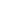Flat 50% off

Ends in

###### Binomial Distribution | CBSE 12th Mathematics in English
927 views###### Binomial Distribution | CBSE 12th Mathematics in English

Published on 05, Aug 2019

Watch video lecture about Binomial Distribution from chapter Probability of Mathematics, online video lecture for CBSE class 12th students, prepared by the best-experienced faculty of Misostudy (https://www.misostudy.com). In probability theory and statistics, the binomial distribution with parameters n and p is the discrete probability distribution of the number of successes in a sequence of n independent experiments, each asking a yes–no question, and each with its own boolean-valued outcome: success/yes/true/one (with probability p) or failure/no/false/zero (with probability q = 1 − p). A single success/failure experiment is also called a Bernoulli trial or Bernoulli experiment and a sequence of outcomes is called a Bernoulli process; for a single trial, i.e., n = 1, the binomial distribution is a Bernoulli distribution. The binomial distribution is the basis for the popular binomial test of statistical significance. In this online video lecture, we will discuss the Theory and Problem Solving of Probability from Mathematics for the preparation of CBSE 2020 exam. Misostudy's courses will help students to boost CBSE preparation to perform best in exams and achieve a good rank.